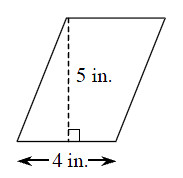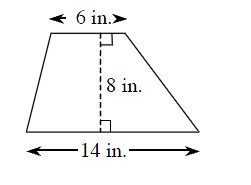### Home > MC2 > Chapter 2 > Lesson 2.3.5 > Problem2-137

2-137.

Use any of your new strategies to find the area of the shapes below. The information in the Math Notes boxes may help. Assume the shape in part (a) is a parallelogram, and the shape in part (b) is a trapezoid. .

1.Form a rectangle using the left portion of the parallelogram. Then find the area of the rectangle.

1.When the trapezoid is copied and rotated, a parallelogram can be formed.

Form a parallelogram using the rotated trapezoid.

Now find the area of the parallelogram.

Use this area to find the area of the original trapezoid.